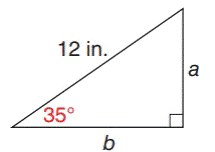Chapter 11.1, Problem 15E### Elementary Geometry for College St...

6th Edition
Daniel C. Alexander + 1 other
ISBN: 9781285195698

#### Solutions

Chapter
Section### Elementary Geometry for College St...

6th Edition
Daniel C. Alexander + 1 other
ISBN: 9781285195698
Textbook Problem
10 views

# In Exercises 15 to 20, find the lengths of the sides named by the variables. Use either Table 11.2 or a calculator, and round answer to the nearest tenth of a unit.To determine

To find:

The length of the side named by the variable.

Explanation

In a right angle triangle,

1) The side PQ, which is opposite to the right angle PRQ is called the hypotenuse.

(The hypotenuse is the longest side of the right triangle.)

2) The side RQ is called the adjacent side of angle θ.

3) The side PR is called the opposite side of angle θ.

Hence, the sine ratio for an acute angle is oppositehypotenuse

Given:

From, the given diagram, opposite side is a, hypotenuse side is 12 in and θ=35

Then,

sin θ=oppositehypotenusesin 35=a12

In a right angle triangle, sum of all three angles is 180

Here, one angle is 90 and other angle is 35

Let the third angle be α

α=1809035α=55

For the angle α, hypotenuse side is 20 m and opposite side is b.

sin α=oppositehypotenusesin 55=b12

Using the table 11

### Still sussing out bartleby?

Check out a sample textbook solution.

See a sample solution

#### The Solution to Your Study Problems

Bartleby provides explanations to thousands of textbook problems written by our experts, many with advanced degrees!

Get Started